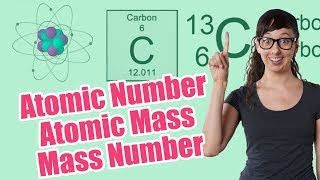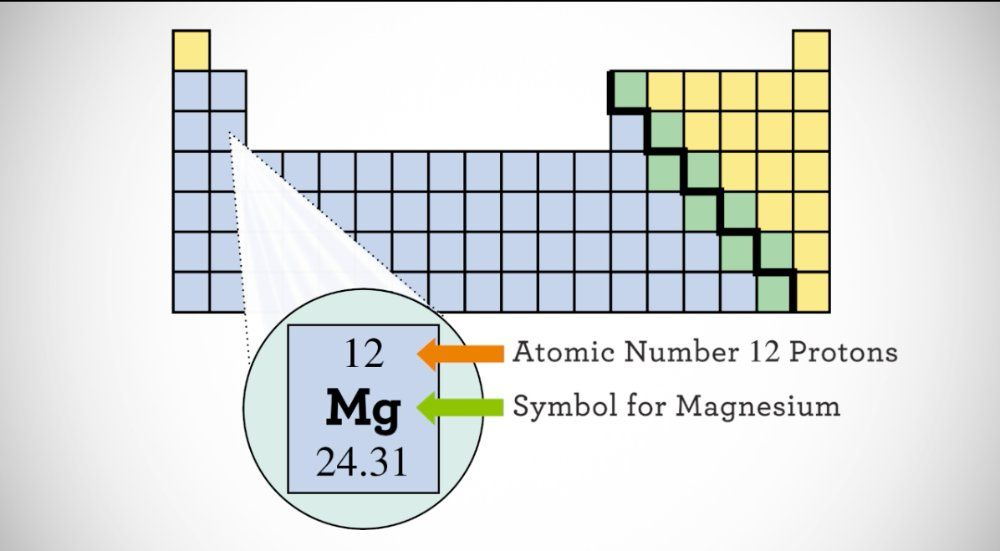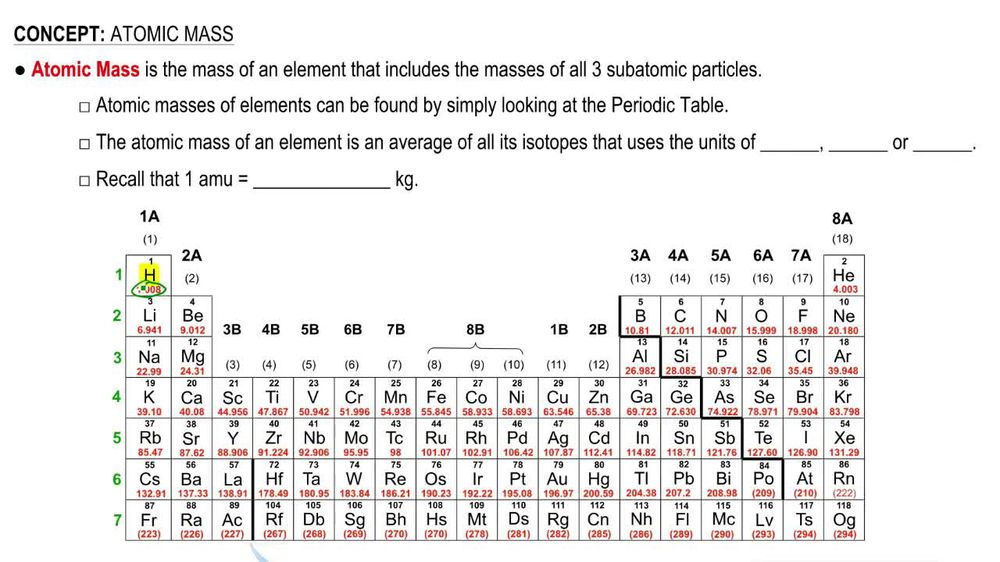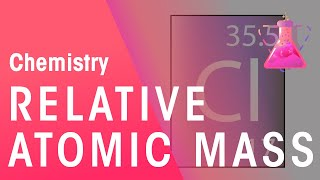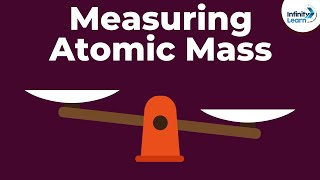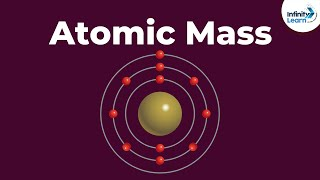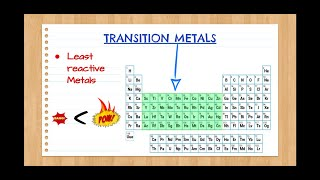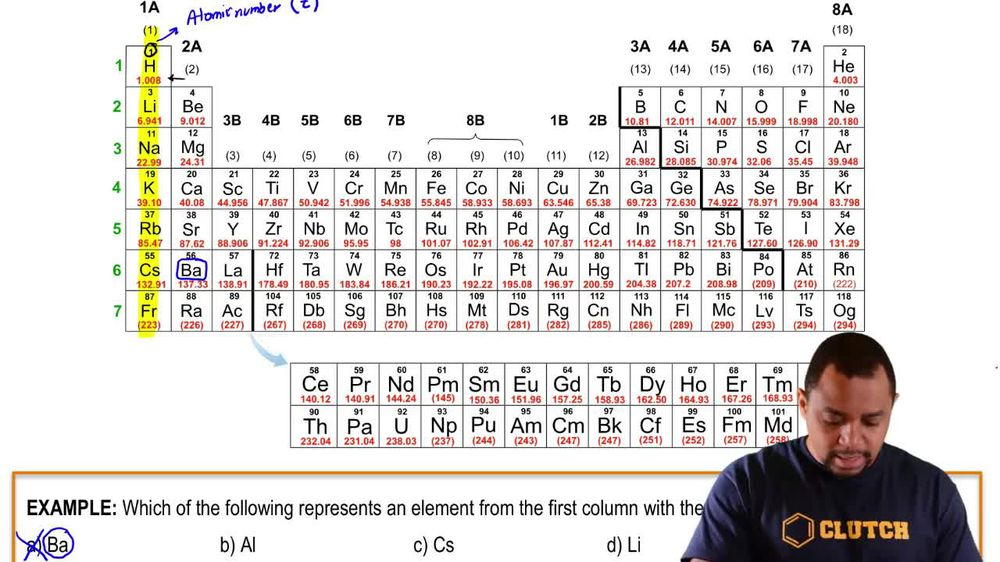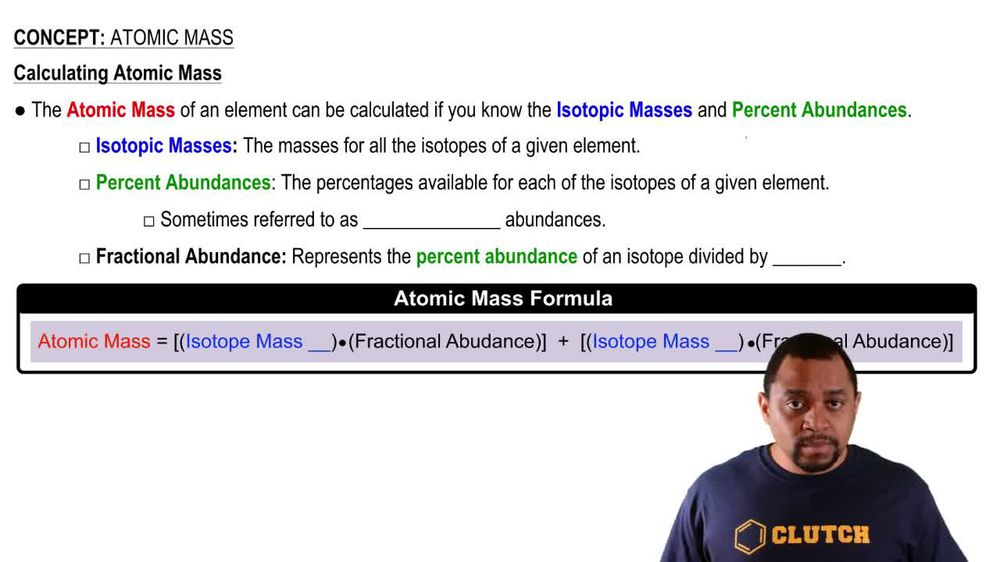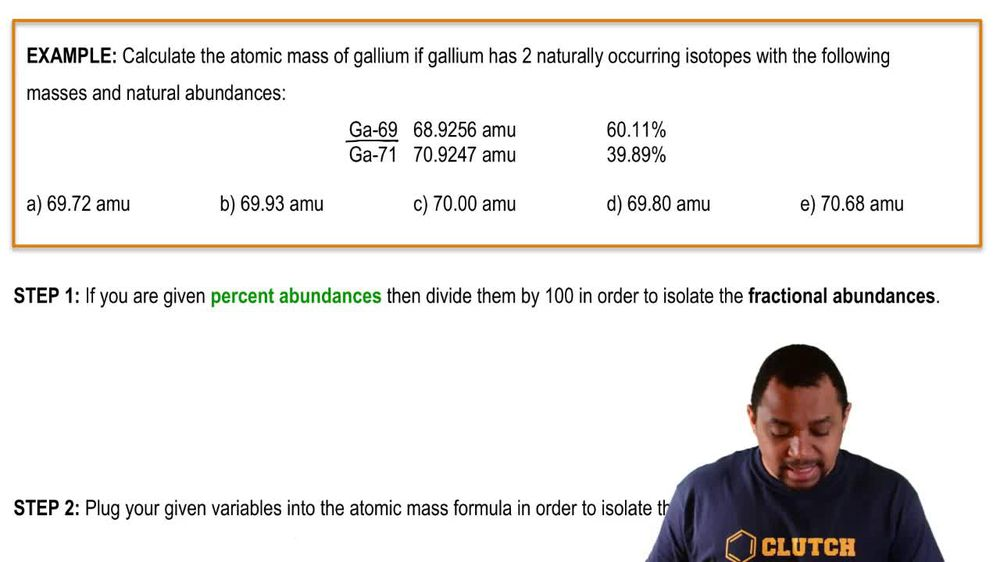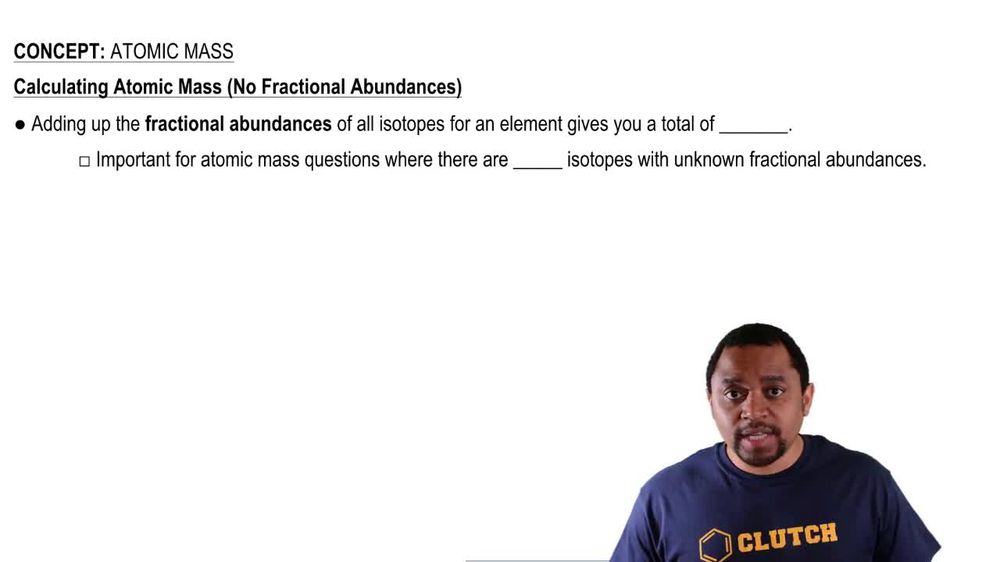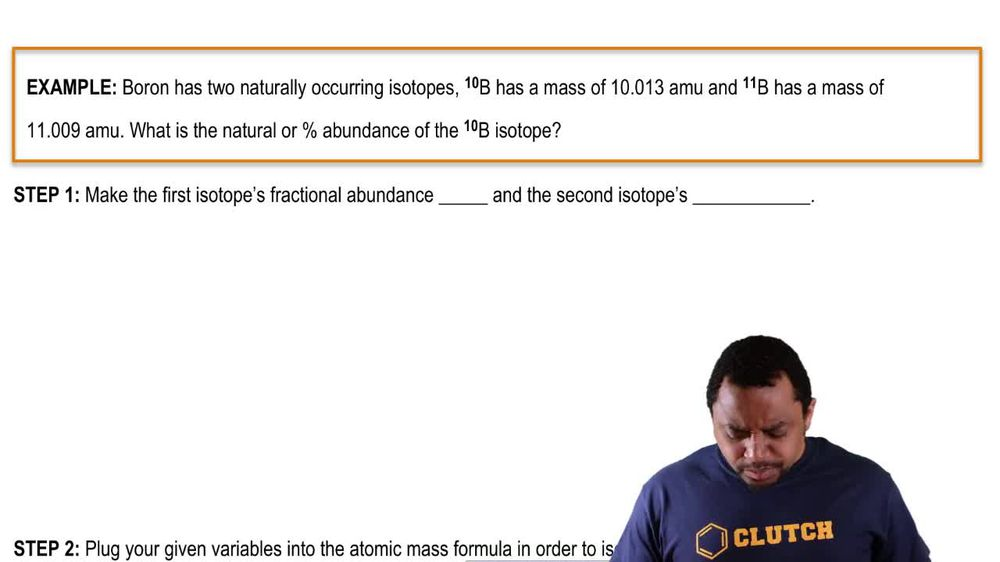Start typing, then use the up and down arrows to select an option from the list.
1. 2. Atoms & Elements2. Atomic Mass# Atomic Mass Example 2

by Jules Bruno
159 views
0
in the following example. Question, it says calculate the atomic mass of gallium if galleon has two naturally occurring isotopes with the following masses and natural abundances. So here we're dealing with gallium 69 71. Their atomic masses are written in terms of atomic mass units, and then here we have 60.11% and 39.89%. Those represent the percent abundance is now to find the atomic mass of our gallium element. Let's follow step one. Step one says, if you are given percent abundances, which we were, we're going to divide them by 100 in order to isolate our fractional abundances. So divide them both y 100. When we do that, we're gonna get our fractional abundances. So then we have these now as our fractional abundances step to plug your given variables into the atomic mass formula in order to isolate the missing variable. So this is just a simple plug and chug algebraic type of situation. Our atomic mass of our element equals the mass of the first isotope times. It's fractional abundance, plus the mass of our second isotope times. It fractional abundance. So when we do that, we're gonna get 69. am you. Since our isotopic masses have four decimal places, we could follow four decimal places here. But we have multiple choice options and we're gonna go with the best answer, which would be option A for this particular question. So using the atomic mass formula is pretty simple. All we have to do is round up all the variables that you're given and isolate the one that's missing. From there, you can find your final answer.